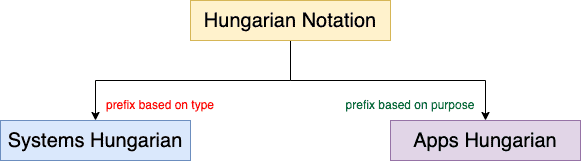## 1. Introduction

In this tutorial, we’ll review naming conventions in computer programming and then delve into Hungarian notation.

## 2. Naming Convention

A naming convention is a collection of rules for naming variables, functions, classes, objects, constants, and other programming identifiers.

A uniform naming convention makes a source code more readable and understandable. It also makes our code clean.

Using it, we can apply standard code quality review tools and get review reports that reveal latent bugs rather than syntax errors. Some popular naming conventions are camel case, snake case, kebab case, and pascal case.

## 3. Hungarian Notation

Charles Simonyi from Microsoft invented the Hungarian notation. Hungarian notation was extensively used in Microsoft Windows code for a few years, starting in 1972. However, it was the era of weakly-typed low-level languages that didn’t do any type-checking at all.

In Hungarian notation, we add a prefix to an identifier that gives information such as its type, length, or scope.

A prefix is typically an abbreviation for the type of a variable or its scope:

• b indicates a boolean
• i stands for an integer
• l denotes a long integer
• str represents a string
• f represents a floating number
• m or fn stands for a function or method
• c is for a class
• o represents an object

For example:

``````iUserAge = 21
strUserFirstName = "John"
strUserLastName = "Cena"
fUserIncome = 1000.50
oCurrentUser = cUser(iUserAge, strUserFirstName, strUserLastName, fUserIncome)``````
We can categorize Hungarian notation into two types based on prefixes:### 3.1. Systems Hungarian

In the case of Systems Hungarian, we base our prefix on the type of the identifier. For example:

•denotes an integer variable for storing a person’s age
• f inshows this is a floating-point number.
•is a boolean flag for a proxy server
• c denotes classes, e.g.,.

### 3.2. Apps Hungarian

In Apps Hungarian notation, a prefix reflects the identifier’s purpose. For example, uf inmeans “user flag”. Similarly:

• us can be the prefix for denoting an unsafe string that is not validated (e.g.,denotes the user input on a web form)
• l is for length (e.g.,can denote the height of the room)
• c can stand for counters (e.g.,denotes the count of retry login attempts)

This was the original form of Hungarian notation, but it was eventually replaced with Systems Hungarian.

## 4. Examples

Let’s check out a few examples.

### 4.1. Variables

In the following Python code snippet, we take the first name, last name, and age of a user and then do some processing using these values. In the end, we print the information:

``````strFirstName = input("Please enter your first name: ")
iAge = int(strAge)
print(f"{strFirstName}-{strLastName} is {iage} years old")``````

Variabletells us that it’s an integer, so we immediately know we can do mathematical operations with it. Similarly,tells us that it is a string variable, so we can’t do any mathematical operations on it.

### 4.2. Functions

We generally use fn as the prefix for a user function:

`````` def fnFactorial(iNum):
if iNum==1:
return iNum
else:
return iNum*fnFactorial(iNum-1)``````

### 4.3. Classes and Methods

We can use the c prefix for classes and m for methods:

``````class cRoom:
def __init__(self, fLength, fWidth):
self.fLength = fLength
self.fWidth = fWidth

# method to calculate area
def mCalculateArea(self):
print(f"Area of Room {self.fLength * self.fWidth}")``````

## 5. Pros and Cons of Hungarian Notation

Hungarian notation is useful for weakly-typed languages such as PHP, where we don’t have string type checking. So, it allows a programmer working on the code to instantly determine the type of a variable whenever it appears. Because of that, we can easily prevent programming errors, such as supplying an argument of the wrong type.

Hungarian notation also enforces consistent and systematic variable naming. In general, we should name our identifiers as per 3c (concise, complete, and correct). This saves a lot of time and effort for the entire team. We can use the additional information from the identifier instead of frequently referring back to its declaration to see its type.

On the other side of the coin, extensive use of Hungarian notation clutters up our source code. Further, we don’t get any additional benefit in the case of a strongly typed language such as Java and Python. This is because compilers smartly catch any mismatch.

Moreover, it makes our code verbose. In most modern languages, the proper solution to include all relevant metadata of an identifier is to inbuild it into the type system. This helps the compiler to properly validate and enforce the rules associated with all operations for that identifier.

Here is a tabular representation of the advantages and disadvantages of using Hungarian notation:

``````

## 6. Conclusion

In this article, we’ve studied Hungarian notation for identifiers in computer programming. Hungarian notation is a convention in which an identifier indicates type, purpose, or scope.

In almost all modern functional or object-oriented programming languages, the compiler encodes the value type and its metadata into the datatype or class rather than into the identifier name. Thus, this notation repeats information violating the DRY (do not repeat) principle of programming.

Most programming companies refrain from using Hungarian notation.